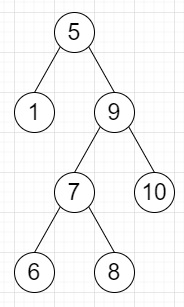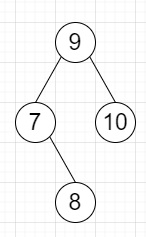# Program to remove all nodes from BST which are not in range in Python

Suppose we have a BST, an two values low and high, we have to delete all nodes that are not between [low, high] (inclusive).

So, if the input is likelow = 7 high = 10, then the output will beTo solve this, we will follow these steps −

• Define a function solve() . This will take root, low, high
• if root is null, then
• return
• if low > data of root, then
• return solve(right of root, low, high)
• if high < data of root, then
• return solve(left of root, low, high)
• right of root := solve(right of root, low, high)
• left of root := solve(left of root, low, high)
• return root

Let us see the following implementation to get better understanding −

## Example

class TreeNode:
def __init__(self, data, left = None, right = None):
self.data = data
self.left = left
self.right = right
def print_tree(root):
if root is not None: print_tree(root.left)
print(root.data, end = ', ') print_tree(root.right)
class Solution:
def solve(self, root, low, high):
if not root:
return
if low > root.data:
return self.solve(root.right,low,high)
if high < root.data:
return self.solve(root.left,low,high)
root.right = self.solve(root.right,low,high)
root.left = self.solve(root.left,low,high)
return root
ob = Solution()
root = TreeNode(5)
root.left = TreeNode(1)
root.right = TreeNode(9) root.right.left = TreeNode(7) root.right.right = TreeNode(10) root.right.left.left = TreeNode(6) root.right.left.right = TreeNode(8) low = 7
high = 10
ret = ob.solve(root, low, high) print_tree(ret)

## Input

root = TreeNode(5)
root.left = TreeNode(1)
root.right = TreeNode(9)
root.right.left = TreeNode(7)
root.right.right = TreeNode(10)
root.right.left.left = TreeNode(6)
root.right.left.right = TreeNode(8)
low = 7
high = 10

## Output

7, 8, 9, 10,